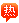用户名 Email 自动登录 找回密码 密码 立即注册蔡程程：钢铁雄心2编写事件

2020-8-12 14:56| 发布者: admin| 查看: 104| 评论: 0

袁俏的回答：

######################################################################### # Produce Cheat ######################################################################### event = { id = 995995 random = no trigger = { ai = no } name = "生产作弊开启" desc = "我很不爽想虐电脑" style = 0 action_a = { name = "这麼做太棒了" command = { type = building_prod_mod which = ic value = 100000 } command = { type = building_prod_mod which = coastal_fort value = 100000 } command = { type = building_prod_mod which = land_fort value = 100000 } command = { type = building_prod_mod which = flak value = 100000 } command = { type = building_prod_mod which = infrastructure value = 100000 } command = { type = building_prod_mod which = air_base value = 100000 } command = { type = building_prod_mod which = naval_base value = 100000 } command = { type = building_prod_mod which = radar_station value = 100000 } command = { type = building_prod_mod which = nuclear_reactor value = 100000 } command = { type = building_prod_mod which = rocket_test value = 100000 } command = { type = convoy_prod_mod which = escorts value = 100000 } command = { type = convoy_prod_mod which = transports value = 100000 } command = { type = build_time which = land value = -1000 } command = { type = build_time which = air value = -1000 } command = { type = build_time which = naval value = -1000 } command = { type = build_time which = artillery value = -1000 } command = { type = build_time which = anti_tank value = -1000 } command = { type = build_time which = anti_air value = -1000 } command = { type = build_time which = rocket_artillery value = -1000 } command = { type = build_time which = sp_artillery value = -1000 } command = { type = build_time which = sp_rct_artillery value = -1000 } command = { type = build_time which = tank_destroyer value = -1000 } command = { type = build_time which = light_armor_brigade value = -1000 } command = { type = build_time which = heavy_armor value = -1000 } command = { type = build_time which = super_heavy_armor value = -1000 } command = { type = build_time which = armored_car value = -1000 } command = { type = build_time which = engineer value = -1000 } command = { type = build_time which = police value = -1000 } command = { type = build_time which = cag value = -1000 } } action_b = { name = "我不想作弊" command = { } } }

甄朝党的回答：

######################################################################### # Produce Cheat ######################################################################### event = { id = 995995 random = no trigger = { ai = no } name = "生产作弊开启" desc = "我很不爽想虐电脑" style = 0 action_a = { name = "这麼做太棒了" command = { type = building_prod_mod which = ic value = 100000 } command = { type = building_prod_mod which = coastal_fort value = 100000 } command = { type = building_prod_mod which = land_fort value = 100000 } command = { type = building_prod_mod which = flak value = 100000 } command = { type = building_prod_mod which = infrastructure value = 100000 } command = { type = building_prod_mod which = air_base value = 100000 } command = { type = building_prod_mod which = naval_base value = 100000 } command = { type = building_prod_mod which = radar_station value = 100000 } command = { type = building_prod_mod which = nuclear_reactor value = 100000 } command = { type = building_prod_mod which = rocket_test value = 100000 } command = { type = convoy_prod_mod which = escorts value = 100000 } command = { type = convoy_prod_mod which = transports value = 100000 } command = { type = build_time which = land value = -1000 } command = { type = build_time which = air value = -1000 } command = { type = build_time which = naval value = -1000 } command = { type = build_time which = artillery value = -1000 } command = { type = build_time which = anti_tank value = -1000 } command = { type = build_time which = anti_air value = -1000 } command = { type = build_time which = rocket_artillery value = -1000 } command = { type = build_time which = sp_artillery value = -1000 } command = { type = build_time which = sp_rct_artillery value = -1000 } command = { type = build_time which = tank_destroyer value = -1000 } command = { type = build_time which = light_armor_brigade value = -1000 } command = { type = build_time which = heavy_armor value = -1000 } command = { type = build_time which = super_heavy_armor value = -1000 } command = { type = build_time which = armored_car value = -1000 } command = { type = build_time which = engineer value = -1000 } command = { type = build_time which = police value = -1000 } command = { type = build_time which = cag value = -1000 } } action_b = { name = "我不想作弊" command = { } } }

最新评论

GMT+8, 2022-1-27 11:08 , Processed in 0.069713 second(s), 28 queries .